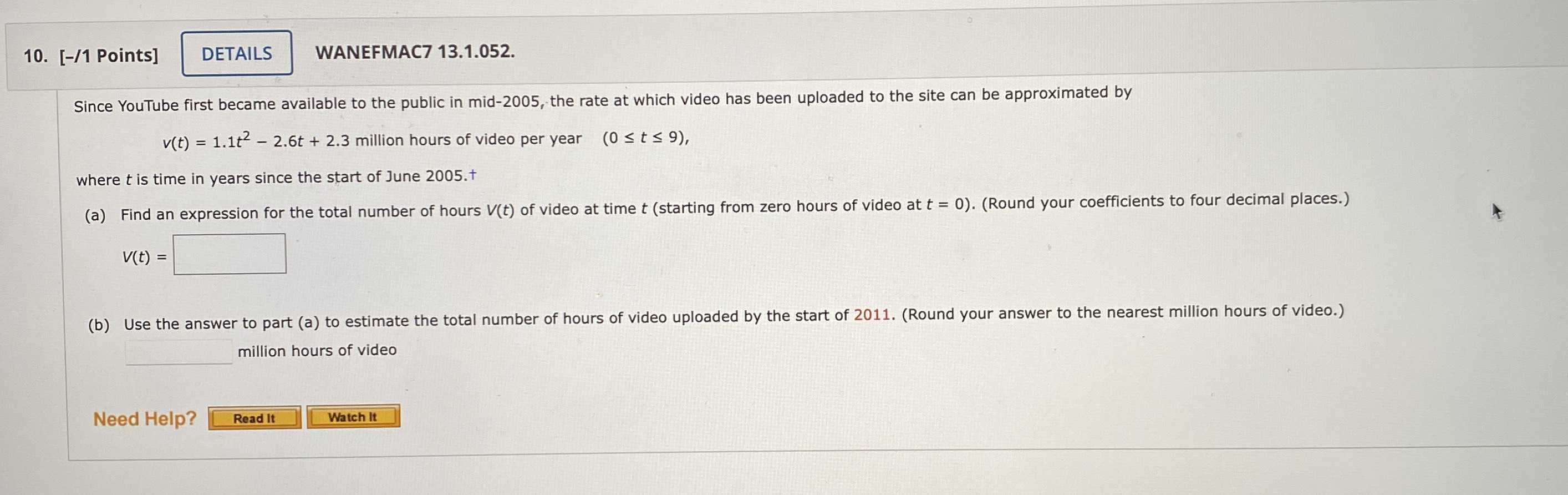### Still have math questions?

Algebra
QuestionSince YouTube first became available to the public in mid-2005, the rate at which video has been uploaded to the site can be approximated by  $$v ( t ) = 1.1 t ^ { 2 } - 2.6 t + 2.3$$ million hours of video per year $$( 0 \leq t \leq 9 )$$ , where $$t$$ is time in years since the start of June $$2005$$ . $$t$$

(a) Find an expression for the total number of hours $$V ( t )$$ of video at time $$t$$ (starting from zero hours of video at $$t = 0$$ ). (Round your coefficients to four decimal place

$$V ( t ) =$$

a) V(t)=$$0.3667t^3- 1.3t^2+ 2.3t$$